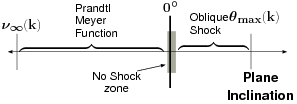# 12.1.1: Preface to Oblique Shock

Fig. 12.1 A view of a straight normal shock as a limited case for oblique shock.

In Section, a discussion on a normal shock was presented. A normal shock is a special type of shock wave. In the literature oblique shock, normal shock, and Prandtl–Meyer function are presented However, one can view all these cases as three different regions of a flow over a plate with a deflection section. Clearly, variation of the deflection angle from a zero ($$\delta = 0$$) to a positive value results in oblique shock (see Figure 12.1). Further changing the deflection angle to a negative value results in expansion waves. The common representation is done by ignoring the boundaries of these models. However, this section attempts to show the boundaries and the limits or connections of these models. A normal shock occurs when there is a disturbance downstream which imposes a boundary condition on the flow in which the fluid/gas can react only by a sharp change in the flow direction. As it may be recalled, normal shock occurs when a wall is straight/flat ($$\delta = 0$$) as shown in Figure 12.1 due to disturbance. When the deflection angle is increased, the gas flow must match the boundary conditions. This matching can occur only when there is a discontinuity in the flow field. Thus, the direction of the flow is changed by a shock with an angle to the flow. This shock is commonly referred to as the oblique shock.Fig. 12.2 The regions where oblique shock or Prandtl–Meyer function exist. Notice that both have a maximum point and a "no solution'' zone, which is around zero.

Decreasing the deflection angle also requires the boundary conditions to match the geometry. Yet, for a negative deflection angle (in this section's notation), the flow must be continuous. The analysis shows that the flow velocity must increase to achieve this requirement. This velocity increase is referred to as the expansion wave. As it will be shown in the next section, as opposed to oblique shock analysis, the increase in the upstream Mach number determines the downstream Mach number and the "negative'' deflection angle. It has to be pointed out that both the oblique shock and the Prandtl–Meyer function have a maximum point for $$M_1 \rightarrow \infty$$. However, the maximum point for the Prandtl–Meyer function is much larger than the oblique shock by a factor of more than 2. What accounts for the larger maximum point is the effective turning (less entropy production) which will be explained in the next chapter (see Figure 12.2).